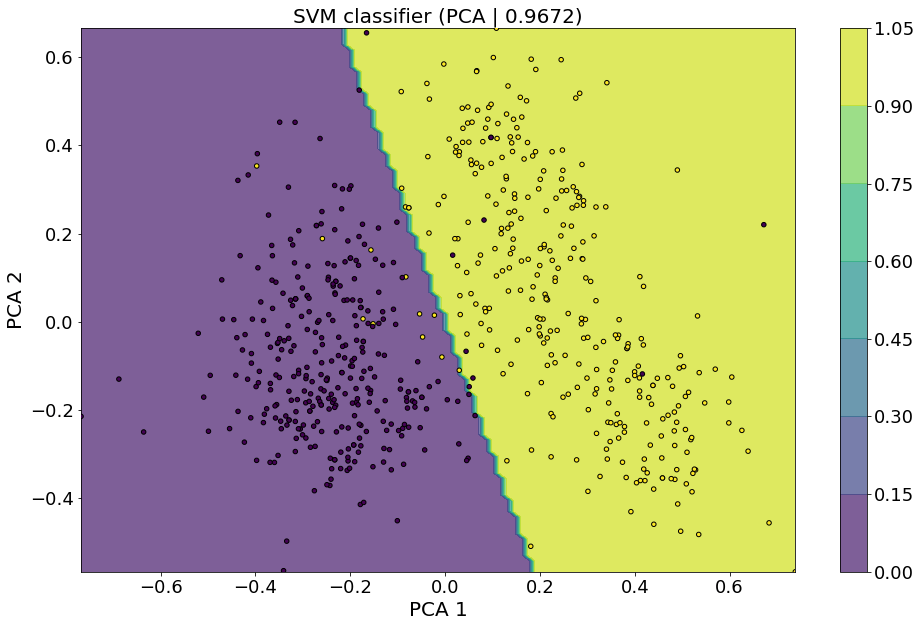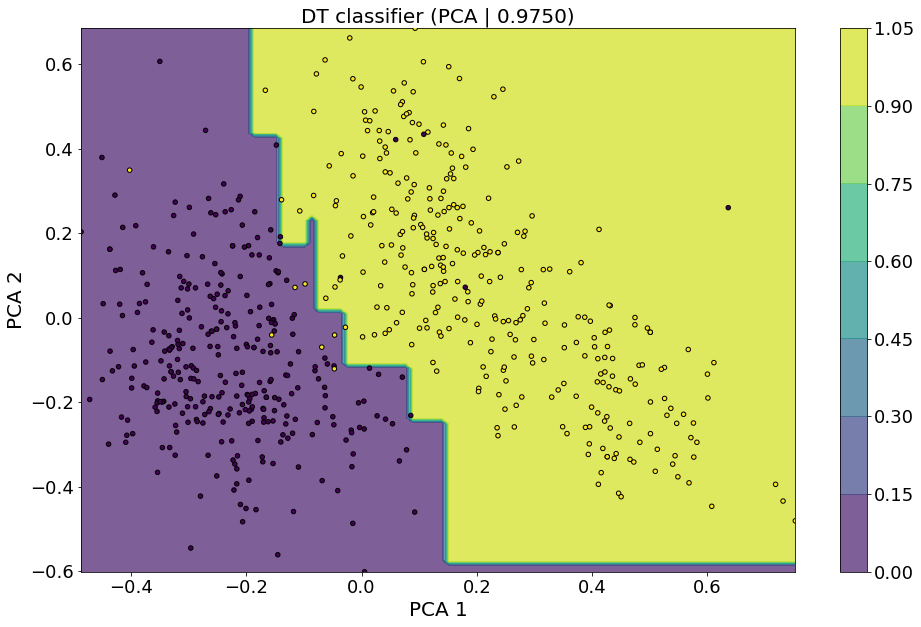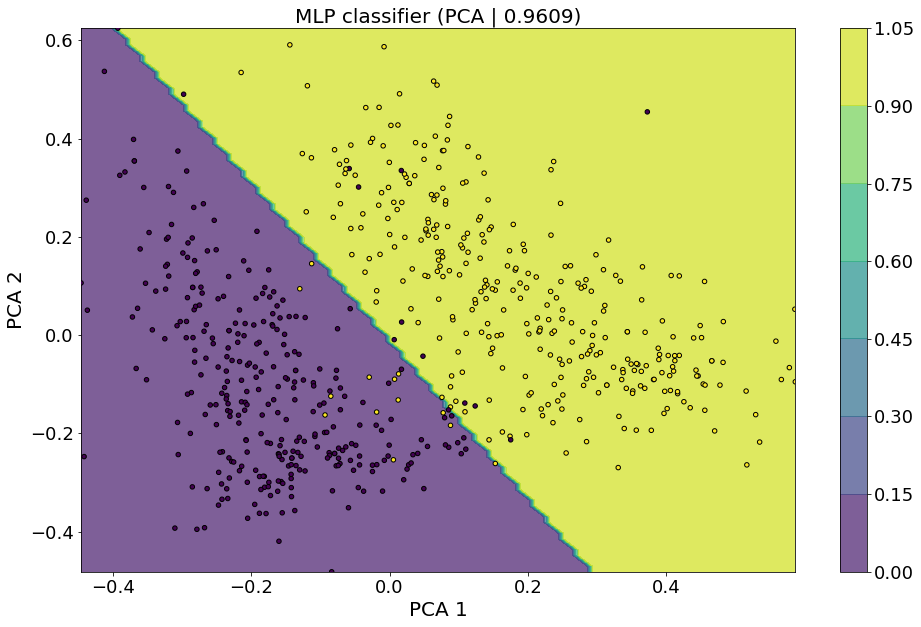`IntroductionData PreprocessingRecursive Feature EliminationPrincipal Component AnalysisHyperparameter TuningSupport Vector MachineK-Nearest NeighborsDecision TreeMulti-Layer PerceptronResultsContributors`

### Introduction

Want to learn more about machine learning? In this post we describe a basic machine learning workflow using many of the tools available in scikit-learn. We use the same wine dataset we used in our other post, which you can find here, which covers basic exploratory data analysis (EDA) of the wine dataset. There we explore the dataset using many useful tools found within the Python ecosystem, including Pandas, Jupyter and Seaborn. You might want to read that post first. Here, we take that EDA to the next level by exploring machine learning (ML) techniques.

We have several goals in mind for this post:

• apply ML to the wine dataset to show what can be done beyond basic EDA,
• describe and compare several ML models,
• examine the ML workflow, including hyperparameter tuning,
• explore dimensionality reduction of the data before using ML models.

To keep things simple, and to exploit many of the useful helper functions, we use scikit-learn as our library. Scikit-learn is a Python library that provides methods for data processing, hyperparameter tuning, and algorithm training and testing. The Jupyter notebook we wrote can be found hereThe wine dataset can be found here. The four ML models we compare are:

Let’s now turn to the ML workflow, starting with processing the data based on the EDA.

Sorry, we cannot display this file.
Sorry, this file is invalid so it cannot be displayed.
view raw wine_UQ.ipynb hosted with ❤ by GitHub

### Preprocessing

The wine dataset has twelve features and a label for red or white; thus, we wish to draw a classification boundary in a twelve dimensional space that separates reds from whites using chemical measurements as the input features. This ML problem is called a “binary classifier”. If you recall our EDA from our previous post, it isn’t clear that this is possible – but, we were only able to examine correlations with 2D plots, not in 12D.

The data set had two important aspects that interfered with the classifier’s ability to learn from the data.

1. The dataset is lop-sided: there are more whites than reds. The classifier will bias itself towards predicting whites because the null hypothesis is shifted from 50/50. (Imagine a dataset with 90% whites – the machine would just learn to always say “white” with little “thinking” involved.)
1. Since we have to down sample, we use recursive feature elimination on different down samples to quantify the best features for classification.
2. The features have numeric values differing by orders of magnitude; such differences are arbitrary because the units of measurement are arbitrary. Having these order of magnitude difference makes it difficult to converge the machine algorithm.

To address (1) we randomly select white wine samples until there is an equal number of red and white wine. This step is called down sampling. To address (2) we wrote a unitize data function that set the minimum value to zero and the maximum value to 1, although scikit learn has similar helper functions.

### Dimensionality Reduction

The wine dataset consists of 6497 samples of wine, each with twelve features and a label specifying if it is a red or white wine. Of these  samples 1599 are red wines and 4898 are white wines. While it may seem that having more features would provide machine learning algorithms more ways to classify data, it is not always the case that having more features is beneficial. Additional features require more resources to store and pass through algorithms and as feature space expands many more samples are needed to have a representative sample of possible features. These issues are known as the curse of dimensionality. To combat this we employ two dimensionality reduction methods: recursive feature elimination .

### Recursive Feature Elimination

Recursive feature elimination (RFE) identifies the best features in your data set by using an external classifier that ranks the importance of each feature and removes the least important one. This processes is repeated until the desired number of features remain.

### Principal Component Analysis

Principal component analysis (PCA) identifies a new set of features that are created from linear combinations of existing features. The newly created features are ranked by the total variance they account for in the data. Unlike RFE which picks the best features from the set of features that were measured, PCA creates new features that may be combinations of existing features.

### Hyperparameter Tuning

Each of the classifiers above have tunable parameters associated with them called hyperparameters (i.e. input options of the classifier). A value for each hyperparameter can be chosen using KFold cross validation. This method involves splitting the data into into K chunks and training the classifier on each set of K-1 chunks and testing on the remaining chunk. This process is repeated for each unique combination of hyperparameters and the set of hyperparameters which correspond to the highest average testing accuracy are used.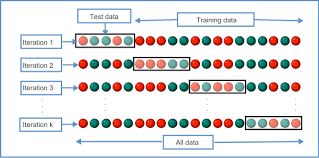### Support Vector Machine

Support vector machines (SVMs) are supervised learning models for both regression and classification.  SVMs were developed at AT&T Bell Laboratories by Vladimir Vapnik in 1992.SVMs can be understood by considering the classification problem shown in the figure. This example has two features X1 and X2 for a binary classification problem with red and blue labels. The dark red classification boundary obviously does not separate well, whereas the dark blue line separates but is shifted toward the light blue points. The optimal, or best, separation is given by the green line, which has maximized the distance from the closest points (those points are the “support vectors”). SVMs can be linear, as shown here, but can also be non-linear using the kernel trick. Because of its optimality, and the ability to use the kernel trick, SVMs are an extremely powerful machine learning method.

Here, we used the
SVM library in scikit-learn, which allows for many options beyond its default settings.  While we explored several options, we ultimately chose the linear kernel because it gave great results very quickly. The only parameter needed is the SVM regularization parameter C, which we varied from [0.1, 1, 10, 100, 1000].

### K-Nearest Neighbors

The second method was nearest neighbors developed by Evelyn Fix and Joesph Hodges in 1951. The technique is given in a declassified document from the USAF school of Aviation Medicine, so it’s original context for development must be inferd.The “nearest neighbors” algorithm applies to both supervised and unsupervised machine learning contexts. We focus on the former with the goal of classifying a new data point (marked as an orange square in the figure) based on the labels of the “closest” points. Definitions of “closeness” vary but common choices include the Manhattan or the Euclidean distance; choosing one defintion over the other may result in a more accurate classifier. The number of neighbors (K) defines how many points (in feature space) are considered to assign a label to the unknown point. Consider the figure, three neighbors corresponds to the dashed circle labeled “K=3”. As there are more points labeled “Class B” in the K=3 circle, the orange square would be classified as “Class B”, the opposite is true for the K=7 circle.

In this case, it is clear that the choice of number of neighbors, and possibly the choice of distance metric, changes the label assigned to the orange square. To find the optimal choice of neighbors, we utilize cross-validation to determine the appropriate number of neighbors and distance metric for the nearest neighbor classifier.

The figure is an example of a two-dimensional feature space. This is useful for visualizing the decision boundary between each class. Often, data exists in a much larger feature space and dimensionality reduction is needed to produce a plot like the figure above. As an example, the wine dataset has twelve features and we explore two methods of dimensionality reduction, highlighting the differences in classification accuracy in two- and three-dimensions.

Here, we used the KNN library  in scikit-learn, which allows for many options beyond its default settings.  While we explored several options, we ultimately chose the ball_tree algorithm because it gave great results very quickly. We additionally varied the number of neighbors between [11-15], weights ‘uniform’ or ‘distance’, and leaf_size in [2,5,10,15].

### Decision TreeThe decision tree (DT) algorithm is a predictive modeling method used in machine learning/data mining. It uses a decision tree to reach conclusions based on input variables. The DT algorithm uses multiple algorithms to decide to split a node into two or more sub-nodes. The goal of the DT algorithm is to obtain a training model that can be used for predicting the value of the target variable by learning decision rules from training data. DTs are an example of white box machine learning, in that they are easily interpreted by humans.

The figure shows the survival of the passengers on the Titanic (‘sibsp’ is the number of spouses or siblings aboard). The figure under the nodes shows the survival probability and percentage of observations of the node. This result shows that the chance of survival is high if you are a female or a male with less than three siblings.

Here, we used the DT library in scikit-learn, which allows for many options beyond its default settings. While we explored several options. We varied the criterion between ‘gini’,’entropy’between [11-15], weights ‘uniform’ or ‘distance’, and leaf_size in [2,5,10,15].

### Multi-layer Perceptron

A multi-layer perceptron (MLP) is most commonly known as a deep neural network. As the name suggests the basic building block is a perceptron (neuron). The linear perceptron was invented by Frank Rosenblatt in 1957. Following from biological inspirations, it was invented with the goal of image classification in mind (as the name ‘perceptron’ suggests).

An MLP has three main components: an input layer, one or more hidden layers, and an output layer. The hidden layers and the output layers are composed of many perceptrons or neurons. The figure below shows an example of a MLP with one hidden layer.The left layer with red circles is the input layer, this is your data {X| x1, …, xn} with n features. The second layer is composed of k neurons (ak), each connected to every input circle, plus one bias neuron. The lines connecting xn to ak represent the weights {w}. Each neuron takes in your data, performs a weighted sum S = w1 x1 + w2 x2 + … , applies a non-linear function f(S) to it, also known as activation function. The result of each neuron is then passed to the next layer of neurons which performs the same actions. This process continues until it reaches the output layer which applies the same function and it returns a value. This value is compared with your labels (target). If the value and the label are different the MLP will change the weights over and over until the label and the return value agree. In other words, the MLP has learned the function.

MLP are used primarily for learning non-linear functions and are useful for real-time learning, that is you can add new data and the MLP will learn without the need to re-train your model.

Here, we used the MLP library in scikit-learn, which allows for many options beyond its default settings.  While we explored several options, we ultimately chose to vary only the width, depth, and activation function of the neural network in order to obtain results in reasonable time.

In our notebook we increase the default number of maximum iterations to 2000, and enabled early stopping. This is because early investigation showed that the default MLP with 1 hidden layer and 100 neurons was not converging with only the default 200 iterations.

### Results

Testing accuracy of classifiers.

 Features/Method Support Vector Machine K-Nearest Neighbors Decision Tree Multi-layer Perceptron RFE with 2 Components 94.9 94.9 94.2 94.6 PCA with 2 Components 96.9 97.1 96.6 96.9 RFE with 3 Components 96.7 97.7 96.8 96.9 PCA with 3 Components 97.0 97.7 96.6 97.1 Full 99.3 99.0 97.4 99.1

Decision boundary of classifiers.

 Features/Method Support Vector Machine K-Nearest Neighbors Decision Tree Multi-layer Perceptron RFE with 2 Components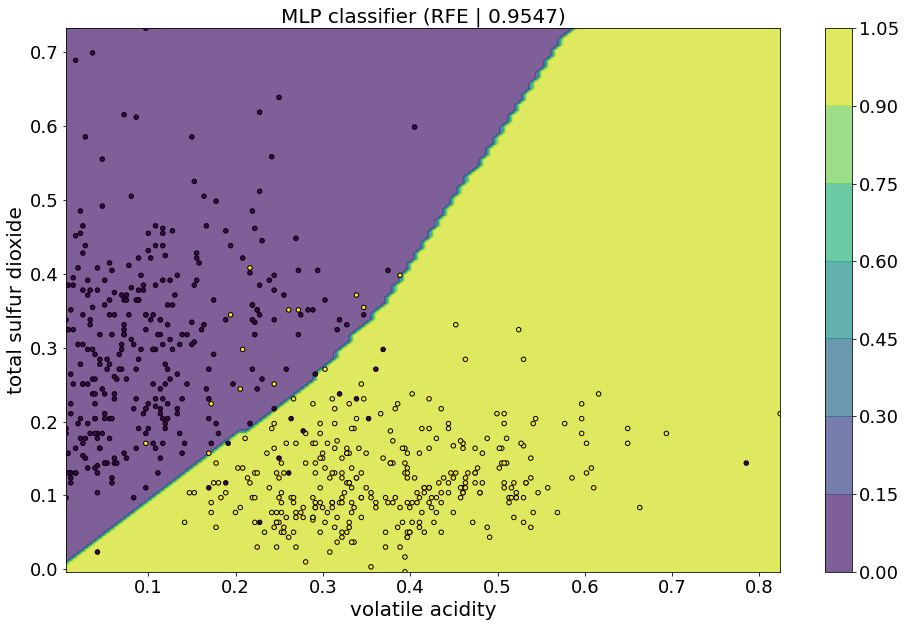PCA with 2 Components